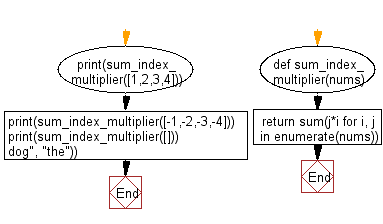﻿ Python: Sum of all items of a given array of integers where each integer is multiplied by its index - w3resource

# Python: Sum of all items of a given array of integers where each integer is multiplied by its index

## Python Basic - 1: Exercise-100 with Solution

Write a Python program to compute the sum of all items in a given array of integers where each integer is multiplied by its index. Return 0 if there is no number.

Sample Solution:

Python Code:

``````def sum_index_multiplier(nums):
return sum(j*i for i, j in enumerate(nums))

print(sum_index_multiplier([1,2,3,4]))
print(sum_index_multiplier([-1,-2,-3,-4]))
print(sum_index_multiplier([]))
``````

Sample Output:

```20
-20
0
```

Pictorial Presentation:Flowchart:Python Code Editor:

Have another way to solve this solution? Contribute your code (and comments) through Disqus.

What is the difficulty level of this exercise?

Test your Programming skills with w3resource's quiz.

﻿